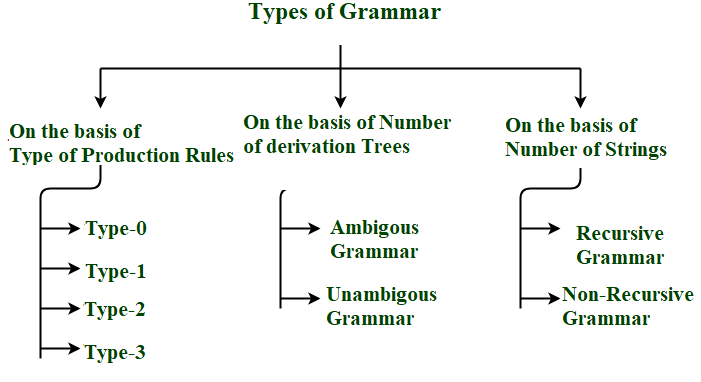Open In App

# Introduction To Grammar in Theory of Computation

Prerequisite – Theory of Computation

Grammar :
It is a finite set of formal rules for generating syntactically correct sentences or meaningful correct sentences.

Constitute Of Grammar :
Grammar is basically composed of two basic elements –

1. Terminal Symbols –
Terminal symbols are those which are the components of the sentences generated using a grammar and are represented using small case letter like a, b, c etc.
2. Non-Terminal Symbols –
Non-Terminal Symbols are those symbols which take part in the generation of the sentence but are not the component of the sentence. Non-Terminal Symbols are also called Auxiliary Symbols and Variables. These symbols are represented using a capital letter like A, B, C, etc.

Formal Definition of Grammar :
Any Grammar can be represented by 4 tuples – <N, T, P, S>

• N – Finite Non-Empty Set of Non-Terminal Symbols.
• T – Finite Set of Terminal Symbols.
• P – Finite Non-Empty Set of Production Rules.
• S – Start Symbol (Symbol from where we start producing our sentences or strings).

Production Rules :
A production or production rule in computer science is a rewrite rule specifying a symbol substitution that can be recursively performed to generate new symbol sequences. It is of the form α->  β where  α is a Non-Terminal Symbol which can be replaced by β which is a string of Terminal Symbols or Non-Terminal Symbols.

Example-1 :
Consider Grammar G1 = <N, T, P, S>

T = {a,b}    #Set of terminal symbols
P = {A->Aa,A->Ab,A->a,A->b,A->}    #Set of all production rules
S = {A}    #Start Symbol

As the start symbol is S then we can produce Aa, Ab, a,b,which can further produce strings where A can be replaced by the Strings mentioned in the production rules and hence this grammar can be used to produce strings of the form (a+b)*.

Derivation Of Strings :

A->a    #using production rule 3
OR
A->Aa    #using production rule 1
Aa->ba    #using production rule 4
OR
A->Aa    #using production rule 1
Aa->AAa    #using production rule 1
AAa->bAa    #using production rule 4
bAa->ba    #using production rule 5

Example-2 :
Consider Grammar G2 = <N, T, P, S>

N = {A}   #Set of non-terminals Symbols
T = {a}    #Set of terminal symbols
P = {A->Aa, A->AAa, A->a, A->}    #Set of all production rules
S = {A}   #Start Symbol

As the start symbol is S then we can produce Aa, AAa, a,which can further produce strings where A can be replaced by the Strings mentioned in the production rules and hence this grammar can be used to produce strings of form (a)*.

Derivation Of Strings :

A->a    #using production rule 3
OR
A->Aa    #using production rule 1
Aa->aa    #using production rule 3
OR
A->Aa    #using production rule 1
Aa->AAa    #using production rule 1
AAa->Aa    #using production rule 4
Aa->aa    #using production rule 3

Equivalent Grammars :
Grammars are said to be equivalent if they produce the same language.

Different Types Of Grammars :
Grammar can be divided on basis of –

• Type of Production Rules
• Number of Derivation Trees
• Number of Strings